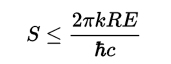# The Physics Equation That Proves I Should Eat More Doughnuts

The Bekenstein Bound:Not to be confused with Broadway Bound.

At any rate, I came across this equation in a book I’m reading on physics. Here’s what Wikipedia says:

In physics, the Bekenstein bound is an upper limit on the entropy S, or information I, that can be contained within a given finite region of space which has a finite amount of energy—or conversely, the maximum amount of information required to perfectly describe a given physical system down to the quantum level. It implies that the information of a physical system, or the information necessary to perfectly describe that system, must be finite if the region of space and the energy is finite. In computer science, this implies that there is a maximum information-processing rate (Bremermann’s limit) for a physical system that has a finite size and energy, and that a Turing machine with finite physical dimensions and unbounded memory is not physically possible.

The universal form of the bound was originally found by Jacob Bekenstein as the inequality where S is the entropy, k is Boltzmann’s constant, R is the radius of a sphere that can enclose the given system, E is the total mass–energy including any rest masses,

To put it more succinctly in a way that I can understand (that is, language that encourages me to eat doughnuts), The maximum amount of information that can be contained increases with mass. Mass/Energy. Whatever.

More doughnuts for me. I must increase gain weight to get smarter.